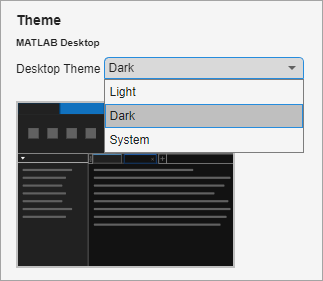## 更改桌面颜色

### 更改文本和背景颜色

1. 主页选项卡上的环境部分中，点击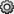2. 选择 MATLAB > 颜色

MATLAB Online 中，选择 MATLAB > 外观 > 颜色

3. 桌面工具颜色部分，清除使用系统颜色复选框。

MATLAB Online 中，使用系统颜色复选框不可用，可以跳过此步骤。

4. 使用文本背景字段更改颜色。例如，选择白色作为文本颜色，黑色作为背景颜色。

语法高亮示例命令行窗口示例区域显示所选颜色预览。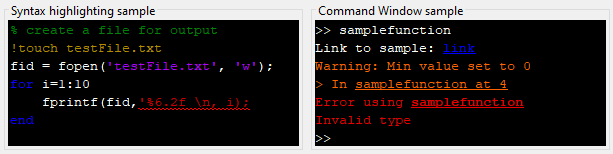要还原默认文本和背景颜色，请点击预设项窗口底部的按钮。

### 更改语法高亮颜色

MATLAB 在代码中使用不同颜色表示语法信息。此功能也称为语法高亮，可帮助您一目了然地识别语法元素，例如 `if/then/else` 语句。语法高亮出现在代码文件、在命令行窗口中输入的代码以及错误和警告消息中。它不会出现在其他类型的输出中。

1. 主页选项卡上的环境部分中，点击2. 选择 MATLAB > 颜色

MATLAB Online 中，选择 MATLAB > 外观 > 颜色

3. MATLAB 语法高亮颜色部分中，使用关键字注释字符串未终止的字符串系统命令语法错误验证节字段更改颜色。例如，选择品红色作为字符串颜色，选择紫色作为未终止的字符串颜色。

语法高亮示例区域显示所选颜色预览。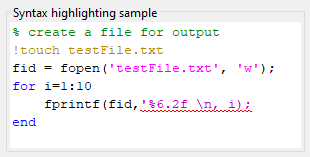要还原默认语法高亮颜色，请点击预设项窗口底部的按钮。

### 更改输出颜色

MATLAB 使用不同颜色来识别命令行窗口和实时编辑器输出中的错误、警告和链接。

1. 主页选项卡上的环境部分中，点击2. 选择 MATLAB > 颜色

MATLAB Online 中，选择 MATLAB > 外观 > 颜色

3. MATLAB 输出颜色部分中，使用错误文本警告文本命令行窗口中的超链接字段更改颜色。例如，选择品红色用于错误文本，绿色用于警告文本。

命令行窗口示例区域显示所选颜色预览。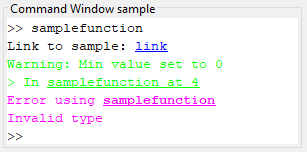要还原默认输出颜色，请点击预设项窗口底部的按钮。

### 更改编程工具颜色

#### 变量和函数颜色

MATLAB 还使用不同颜色的文本在编辑器和实时编辑器中标注具有共享作用域的变量。要更改具有共享作用域的变量的颜色，请在变量和函数颜色部分中，从具有共享作用域的变量字段中选择一种颜色。要禁止在编辑器和实时编辑器中标注具有共享作用域的变量，请清除具有共享作用域的变量复选框。有关具有共享作用域的变量的详细信息，请参阅在编辑器中检查变量作用域

### 在 MATLAB Online 中选择主题

MATLAB Online 中，您还可以使用主题来更改 MATLAB 桌面的颜色。MATLAB 的安装版本不支持主题。

“MATLAB 外观预设项”页会显示所选主题的预览。